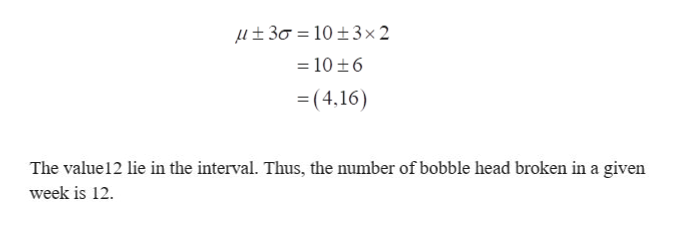Question
64 views

3. The number of broken bobble heads found on a bobble head production line every week follows a normal distribution with a mean of 10 bobble heads and a standard deviation of 2. Use the emperical rule and/or symmetry to justify your answers.

A. What number of bobble heads broken in a given week would be considered the 84th percentile? Use the empircal rule and/or symmetry to justify answers.

B. Would youu be surprised if, in a given week, less than 10 bobble heads were broken? Justify your answer. Use the empirical rule/and or symmetry to justify answers.

C. What range of weekly broken bobble heads would occur 95% of the time. Use the empirical rule and/or symmetry to justify answers.

check_circle

Step 1

From the provided information, the mean (µ) and standard deviation (σ) are 10 and 2 respectively.

A.   Let the number of bobble heads broken in a given week is x. The z score at 84th percentile from the z score table is 1.

Now, the required number of bobble can be obtained as;

Step 2

Using the empirical rule, 99.7% of the data lie within three standard deviation from the mean which can be obtained as;help_outlineImage Transcriptionclose30 10+3 x2 = 10 +6 =(4,16) The value 12 lie in the interval. Thus, the number of bobble head broken in a given week is 12 fullscreen
Step 3

B.  It would not be surprised if, in a given week less than 10 bobble heads were broken because the interval which contain...

### Want to see the full answer?

See Solution

#### Want to see this answer and more?

Solutions are written by subject experts who are available 24/7. Questions are typically answered within 1 hour.*

See Solution
*Response times may vary by subject and question.
Tagged in

### Other